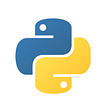# Calculate a Number Factorial in Python — pythonpip.com

This python tutorial help to calculate factorial using Numpy and without Numpy. The factorial is always computed by multiplying all numbers from 1 to the number given. The factorial is always found for a positive integer.

in python, we can calculate a given number factorial using loop or math function, I’ll discuss both ways to calculate factorial for a number in python.

# Using for Loop

We can use a for loop to iterate through number 1 until we get to the given number, multiplying each time.

`n = input("Enter a number: ") factorial = 1 if int(n) >= 1: for i in range (1,int(n)+1): factorial = factorial * i print("Factorail of ",n , " is : ",factorial)`

Output:

`Enter a number: 4 Factorial of 4 is : 24`

We can also calculate factorial using recursively way.

`num = input("Enter a number: ") def fact_recursive(n): if n == 1: return n elif n < 1: return ("NA") else: return n*fact_recursive(n-1) print("The factorial of ", num, " is : ") print (fact_recursive(int(num)))`

Output:

`Enter a number: 5 The factorial of 5 is : 120`

# Calculate Factorial Using math.factorial()

The factorial function in the math module can be used directly. We can utilize `math.factorial` instead of writing the code for factorial functionality. Negative and fractional numbers are likewise taken care of in this way.

`import math num = input("Enter a number: ") print("The factorial of ", num, " is : ") print(math.factorial(int(num)))`

Output:

`Enter a number: 5 The factorial of 5 is : 120`

# Factorial of an integer with NumPy in Python

We can also calculate a number factorial using NumPy library. The `numpy.math.factorial()` method is used to calculate the factorial of a number.

`import numpy as np num = input("Enter a number: ") factorial = np.math.factorial(int(num)) print("Factorail of ",num , " is : ",factorial)`

Output:

`Enter a number: 6 Factorail of 6 is : 720`

Originally published at https://www.pythonpip.com on January 8, 2022.

--

--

--

## More from Parvez Alam

Hey, I am Parvez Alam. A software developer since 2009. I am a programming addict. I love learning and sharing knowledge. https://www.phpflow.com/

Love podcasts or audiobooks? Learn on the go with our new app.## Parvez Alam

Hey, I am Parvez Alam. A software developer since 2009. I am a programming addict. I love learning and sharing knowledge. https://www.phpflow.com/

## Data Types in Python